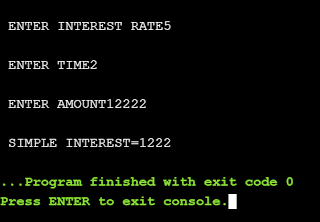## C Program to find Simple Interest in Language C

### welcome back to codejunction.in , hope you have a good time reading our blogs.

THIS PROGRAM IS GOING TO CALCULATE SIMPLE INTEREST . THE USER NEEDS TO INPUT THE INTEREST RATE , TIME AND AMOUNT AND THEN SIMPLE INTEREST WILL BE CALCULATED.

This C Program calculates the simple interest given the principal amount, rate of interest and time. The formula to calculate the simple interest is: simple_interest = (p * t * r) / 100 where p is principal amount, t is time & r is rate of interest.

### CODE

#include<stdio.h>

int main()
{
int i,a,t,si;
printf("\n ENTER INTEREST RATE");
scanf("%d",&i);

printf("\n ENTER TIME");
scanf("%d",&t);

printf("\n ENTER AMOUNT");
scanf("%d",&a);

si=a*i*t/100;
printf("\n SIMPLE INTEREST=%d",si);
}

OUTPUT